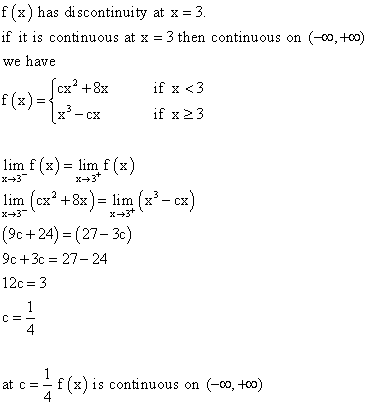# For what value of the constant c is the function f continuous on (−∞ ∞)

For what value of the constant c is the function f continuous on
(-infinity, infinity)? f(x) =cx2 + 8x if
x < 3 cx 2 x x < 3 =x3? cx if x ? 3 = x 3 ? cx if x ? 3

cx2 + 8x =
x3 ? cx
put x=3
9c+24 = 27 – 3c
c=1/4
f(3-) = c*32 + 8*3 = 9c+24
f(3+)= 33-3c = 27-3c
to make the function countinous f(3-) =
f(3+)
9c+24 =27-3c
12c= 3
c= 1/4